# Derivative

## Derivative rules

Derivative rules and laws. Derivatives of functions table.

### Derivative definition

The derivative of a function is the ratio of the difference of function value f(x) at points x+Δx and x with Δx, when Δx is infinitesimally small. The derivative is the function slope or slope of the tangent line at point x.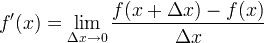### Second derivative

The second derivative is given by: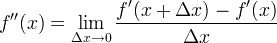Or simply derive the first derivative: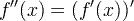### Nth derivative

The nth derivative is calculated by deriving f(x) n times.

The nth derivative is equal to the derivative of the (n-1) derivative:

f (n)(x) = [f (n-1)(x)]'

#### Example:

Find the fourth derivative of

f (x) = 2x5

f (4)(x) = [2x5]'''' = [10x4]''' = [40x3]'' = [120x2]' = 240x

### Derivative on graph of function

The derivative of a function is the slop of the tangential line.

### Derivative rules

 Derivative sum rule ( a f (x) + bg(x) ) ' = a f ' (x) + bg' (x) Derivative product rule ( f (x) ∙ g(x) ) ' = f ' (x) g(x) + f (x) g' (x) Derivative quotient rule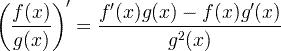Derivative chain rule f ( g(x) ) ' = f ' ( g(x) ) ∙ g' (x)

### Derivative sum rule

When a and b are constants.

( a f (x) + bg(x) ) ' = a f ' (x) + bg' (x)

#### Example:

Find the derivative of:

3x2 + 4x.

According to the sum rule:

a = 3, b = 4

f(x) = x2 , g(x) = x

f ' (x) = 2x , g' (x) = 1

(3x2 + 4x)' = 3⋅2x+4⋅1 = 6x + 4

### Derivative product rule

( f (x) ∙ g(x) ) ' = f ' (x) g(x) + f (x) g' (x)

### Derivative quotient rule### Derivative chain rule

f ( g(x) ) ' = f ' ( g(x) ) ∙ g' (x)

This rule can be better understood with Lagrange's notation: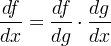### Function linear approximation

For small Δx, we can get an approximation to f(x0+Δx), when we know f(x0) and f ' (x0):

f (x0x) ≈ f (x0) + f '(x0)⋅Δx

### Derivatives of functions table

Function name Function Derivative

f (x)

f '(x)
Constant

const

0

Linear

x

1

Power

x a

a x a-1

Exponential

e x

e x

Exponential

a x

a x ln a

Natural logarithm

ln(x)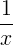Logarithm

logb(x)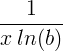Sine

sin x

cos x

Cosine

cos x

-sin x

Tangent

tan x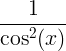Arcsine

arcsin x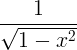Arccosine

arccos x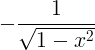Arctangent

arctan x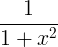Hyperbolic sine

sinh x

cosh x

Hyperbolic cosine

cosh x

sinh x

Hyperbolic tangent

tanh x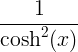Inverse hyperbolic sine

sinh-1 x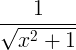Inverse hyperbolic cosine

cosh-1 x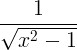Inverse hyperbolic tangent

tanh-1 x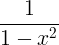### Derivative examples

#### Example #1

f (x) = x3+5x2+x+8

f ' (x) = 3x2+2⋅5x+1+0 = 3x2+10x+1

#### Example #2

f (x) = sin(3x2)

When applying the chain rule:

f ' (x) = cos(3x2) ⋅ [3x2]' = cos(3x2) ⋅ 6x

### Second derivative test

When the first derivative of a function is zero at point x0.

f '(x0) = 0

Then the second derivative at point x0 , f''(x0), can indicate the type of that point:

 f ''(x0) > 0 local minimum f ''(x0) < 0 local maximum f ''(x0) = 0 undetermined

Currently, we have around 1975 calculators, conversion tables and usefull online tools and software features for students, teaching and teachers, designers and simply for everyone.